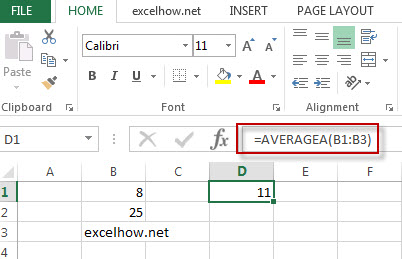# Excel AverageA Function

This post will guide you how to use Excel AVERAGEA function with syntax and examples in Microsoft excel.

### Description

The Excel AVERAGEA function returns the average of its arguments, including numbers, text, and logical values.

The AVERAGEA function is a build-in function in Microsoft Excel and it is categorized as a Statistical Function.

The AVERAGEA function is available in Excel 2016, Excel 2013, Excel 2010, Excel 2007, Excel 2003, Excel XP, Excel 2000, Excel 2011 for Mac.

### Syntax

The syntax of the AVERAGEA function is as below:

= AVERAGEA (value1,[value2],…)

Where the AVERAGEA function arguments are:
Value1 -This is a required argument.  The first values, it can be numbers, Text, logical values, ranges.
Number2 – This is an optional argument.

### Example

The below examples will show you how to use Excel AVERAGEA Function to return the average of the values provided.

#1 To get the average of the numbers in cell range B1:B2, and the text “excelhow.net” in cell B3, just using the following excel formula: =AVERAGEA(B1:B3)